x

# Drafting Workshop in Discrete Mathematics and Probability

2022.01.30. - 2022.02.03.
Erdős Center

### Description

PROGRAM

January 31, 2022 Monday

10:00 - 10:30 Registration

10:30 - 11:10 Cedric Koh: An Accelerated Newton-Dinkelbach Method and its Application to Two Variables Per Inequality Systems

Abstract: In this talk, I will present an accelerated, or 'look-ahead' version of the Newton-Dinkelbach method. Using the Bregman divergence as a potential in conjunction  with combinatorial arguments, we obtain new strongly polynomial algorithms for various problems. In particular, we obtain an improved convergence bound for linear fractional combinatorial optimization, and a strongly polynomial label-correcting algorithm for solving linear feasibility systems with two variables per inequality (2VPI).

11:20 - 12:00 Riley Thornton:  Descriptive Combinatorics and Computer Science

Abstract: I will sketch some recent research connecting descriptive combinatorics to LOCAL algorithms and to constraint satisfaction problems.

12:00 - 14:00 Lunch break

14:00 - 14:40 Partha Pratim Ghosh: A Last Progeny Modified Branching Random Walk

Abstract : In this work, we consider a modification of the usual Branching Random Walk (BRW), where we give certain independent and identically distributed (i.i.d.) displacements to all the particles at the n-th generation, which may be different from the driving increment distribution. We call this process a last progeny modified branching random walk (LPM-BRW). Depending on the value of a parameter, θ, we classify the model in three distinct cases, namely, the boundary case, below the boundary case, and above the boundary case. Under very minimal assumptions on the underlying point process of the increments, we show that at the boundary case, when θ takes a particular value θ0, the maximum displacement converges to a limit after only an appropriate centering, which is of the form c1n − c2logn. We give an explicit formula for the constants c1 and c2 and show that c1 is exactly the same, while c2 is 1/3 of the corresponding constants of the usual BRW. We also characterize the limiting distribution. We further show that below the boundary (that is, when θ <  θ0), the logarithmic correction term is absent. For above the boundary case (that is, when θ >  θ0), we have only a partial result, which indicates a possible existence of the logarithmic correction in the centering with exactly the same constant as that of the classical BRW. For θ ≤ θ0, we further derive Brunet--Derrida-type results of point process convergence of our LPM-BRW to a decorated Poisson point process. Similar results have been obtained also for the time inhomogeneous setting. Surprisingly, the limits in this case depend only on the increments in the initial fraction of time. Under very minimal assumptions, we also derive the large deviation principle (LDP) for the right-most position of a particle in generation n. Our proofs are based on a novel method of coupling the maximum displacement with a linear statistics associated with a more well-studied process in statistics, known as the smoothing transformation.

14:50 - 15:30 John Fernley: Targeted Immunisation Thresholds for the Contact Process on Power-Law Trees

Abstract: The popular scale-free configuration model of a social network has a local limit primarily a Galton-Watson tree with some power law offspring distribution, which has tail exponent parameter 𝛕 greater than 2. It is known that contact process epidemics can propagate on these trees and therefore these networks for arbitrarily small infection rates, and uniformly immunising a positive proportion of vertices in the network will have no effect on this result.
To reduce the possibility of survival of the contact process on these trees we instead remove (immunise) those with largest degree. For small infection rates we find sharp thresholds in the maximum permissible degree for the survival probability through three distinct 𝛕 regimes, which by duality would correspond to a transition in the metastable density of the associated network after targeted removal.

16:00 -        Problem session

February 1, 2022 Tuesday

10:00 - 10:30 Informal session

10:30 - 11:10 Amedeo Sgueglia: Spanning subgraphs in randomly perturbed graphs

Abstract: We study the model of randomly perturbed dense graphs, which is the union of any n-vertex graph G alpha with minimum degree at least alpha n and the binomial random graph G(n,p).
In this talk, we discuss questions on the containment of specific spanning structures, and we offer several new sharp and stability results. We also point out a number of interesting questions that remain open.

This is joint work with Julia Böttcher, Olaf Parczyk, and Jozef Skokan.

11:20 - 12:00 Gábor Damásdi: Coloring geometric hypergraphs

Abstract: I will present some new results, ideas and constructions related to coloring geometric hypergraphs that we have developed with Dömötör Pálvölgyi during my doctoral studies. These will allow us to show the following two results. Firstly we prove that for any planar convex body 𝐶 there is a positive integer 𝑚 with the property that any finite point set 𝑃 in the plane can be three-colored such that there is no translate of 𝐶 containing at least 𝑚 points of 𝑃, all of the same color. The proof relies on a surprising connection to two other famous results, the solution of the two dimensional case of the Illumination conjecture, and a recent solution of the Erdős-Sands-Sauer-Woodrow conjecture by Bousquet, Lochet and Thomassé. In fact, we need a generalization of the latter result.  Secondly we show a complementary result for homotets of convex sets. For every positive integer 𝑚 there is a finite point set 𝒫 in the plane such that no matter how 𝒫 is three-colored, there is always a (not necessarily unit) disk containing exactly 𝑚 points, all of the same color. These theorems improve previous results of Pach, Tardos, Tóth and Pálvölgyi and are sharp in the number of the colors.

12:00 - 14:00 Lunch break

14:00 - 14:40 James Davies: Ringel's circle problem

Abstract:A constellation is a finite collection of circles in the plane in which no three circles are tangent at the same point. In 1959, Ringel asked how many colours are required to colour the circles of a constellation so that tangent circles receive different colours. We present a solution to Ringel's circle problem by constructing constellations that require arbitrarily many colours.

Joint work with Chaya Keller, Linda Kleist, Shakhar Smorodinsky, and Bartosz Walczak.

14:50 - 15:20 Béla Rácz. TBA

18:00 - 18:40 Nóra Frankl - Zoom - Max-norm Ramsey Theory

Abstract: For a subset S of R^d the chromatic number chi(S,R^n) is the minimum number of colours needed to colour all points of R^n without a monochromatic isometric copy of S. In Euclidean Ramsey theory a set S is called Ramsey, if chi(S,R^n) tends to infinity. Classifying Euclidean Ramsey sets is a very difficult open problem. Recently, Kupavskii and Sagdeev showed that if we replace the Euclidean norm with the max norm, then every set is Ramsey. In this talk, we discuss several related results. We find essentially tight bounds for the max-norm chromatic number for certain families of sets. We also discuss related results about covering the integers with translates of given sets, and in particular we prove a conjecture of  Schmidt and Tuller. Joint work with Andrey Kupavskii and Arsenii Sagdeev.

February 2, 2022 Wednesday

Discussions with the PIs

February 3, 2022 Thursday

Discussions with the PIs

Several postdoc positions are available in Budapest in various research groups in discrete mathematics and probability theory. The research groups are listed below.

To make the application process more efficient and joyful, we are organizing a drafting workshop for young researchers interested in these positions, with the dates Jan 31 – Feb 3, 2022, at the Renyi Institute.

The participants of the workshop can present their work in a research talk, learn more about the research environment in Budapest, meet the heads and members of the research groups in person and in case of a perfect matching, get an offer on the spot.

The application due date is December 15th. The workshop is invitation based. We can cover the accommodation of successful applicants and there is limited funding for travel expenses. If you need travel funding, let us know in your application.

A CV containing a publication list should be sent as a part of the application. Recommendation letters are not required, but can be submitted as well. We may contact your PhD advisor asking for more information, so please add their email contacts to the application.

Applications should be sent to the e-mail address large.networks@renyi.hu. Please include 'Drafting workshop application' in the subject of the email. In case the applicant is still a PhD student, the e-mail should contain the expected termination date of their PhD studies.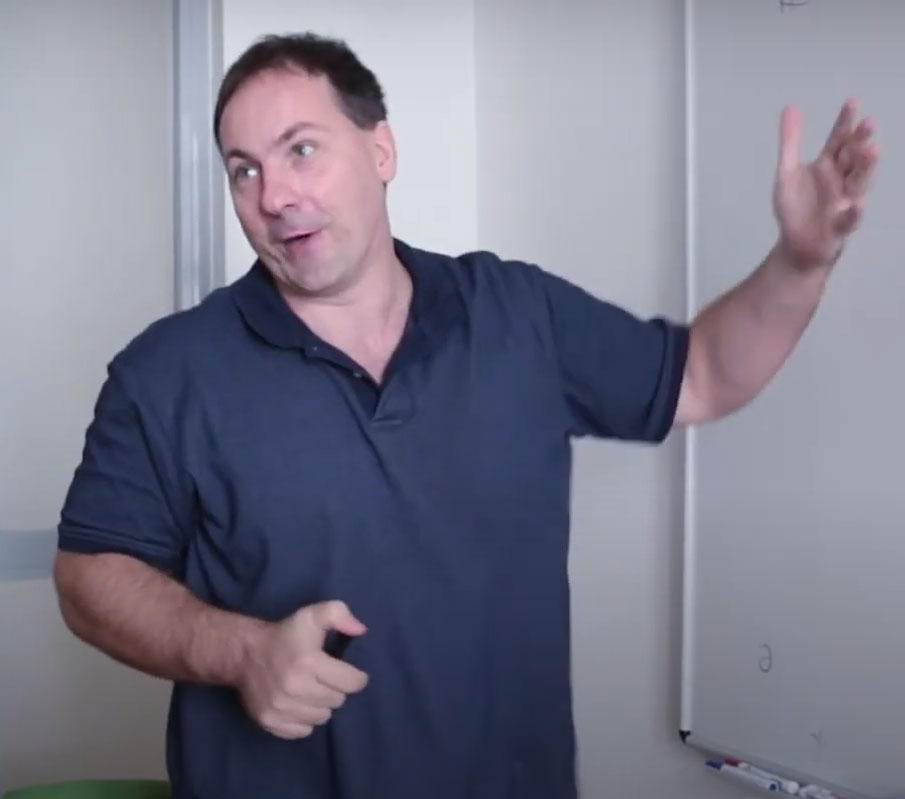Groups and Graph Convergence Research Group

Head: Miklós Abért, Alfréd Rényi Institute of Mathematics

The research group studies measured and asymptotic group theory, in particular spectral theory of graphs and groups, Benjamini-Schramm convergence, stochastic processes on groups, rank gradient, invariant random subgroups, homology growth, sofic entropy and asymptotic invariants of random graphs and locally symmetric spaces.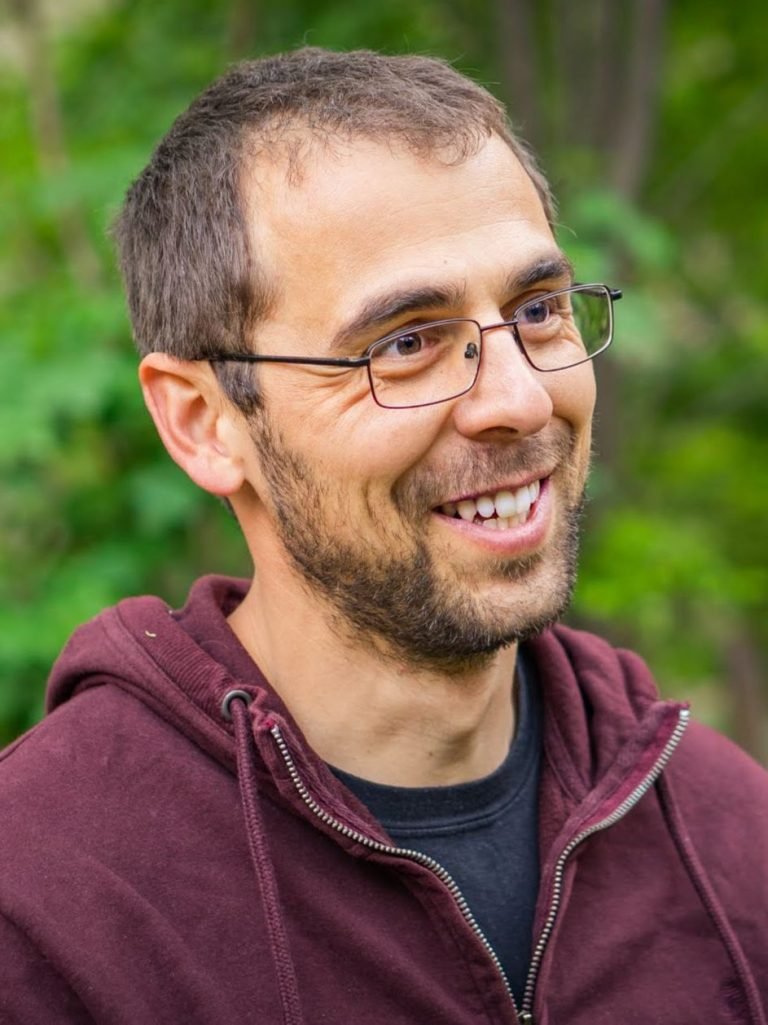MTA-ELTE Momentum Matroid Optimization Research Group

Head: Kristóf Bérczi, Eötvös Loránd University

We aim to explore the global structure of bases in matroids, or more generally, of common bases in the intersection of matroids. Our goal is to devise structural and algorithmic results that will open up new domains for packing common bases algorithmically beyond the currently known special cases. Meanwhile, supported by the results obtained, we aim to resolve a wide list of fundamental open questions that all stem from the global relations of matroid bases.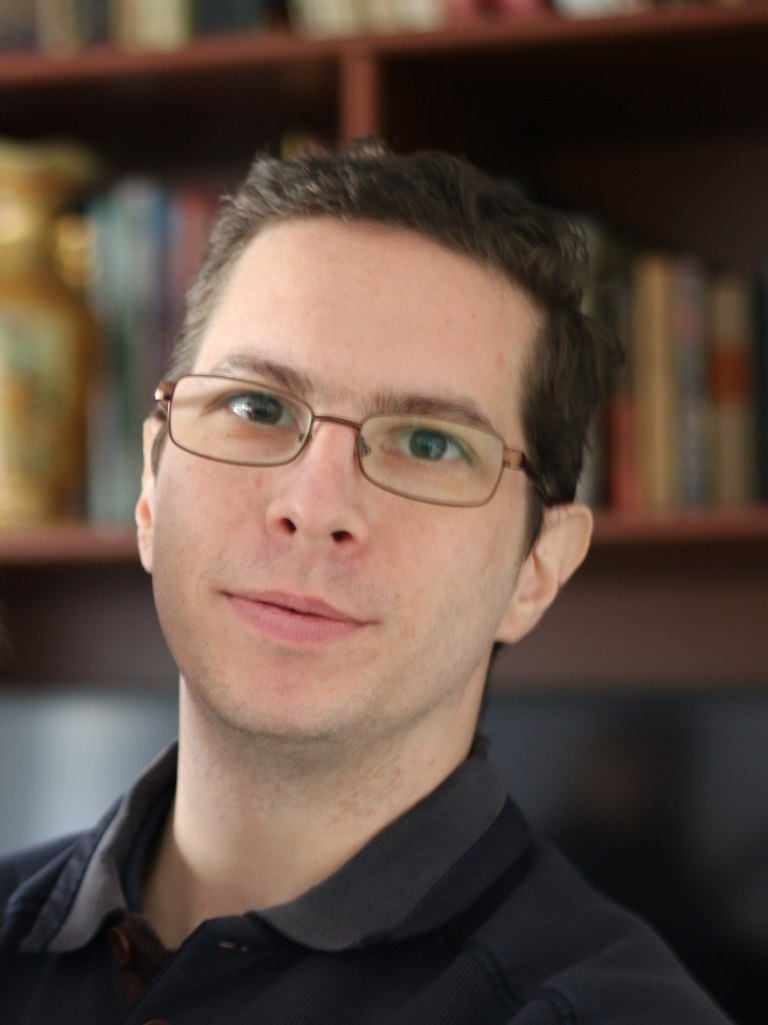MTA-Rényi Momentum Counting in Sparse Graphs Research Group

Head: Péter Csikvári, Alfréd Rényi Institute of Mathematics

We aim to study approximate counting problems of combinatorial objects of a given graph besides restricted information. This general framework includes counting matchings, independent sets, colorings given the degree sequence or local statistics or may be the whole graph as an input. There is an emphasis on developing random and deterministic algorithms.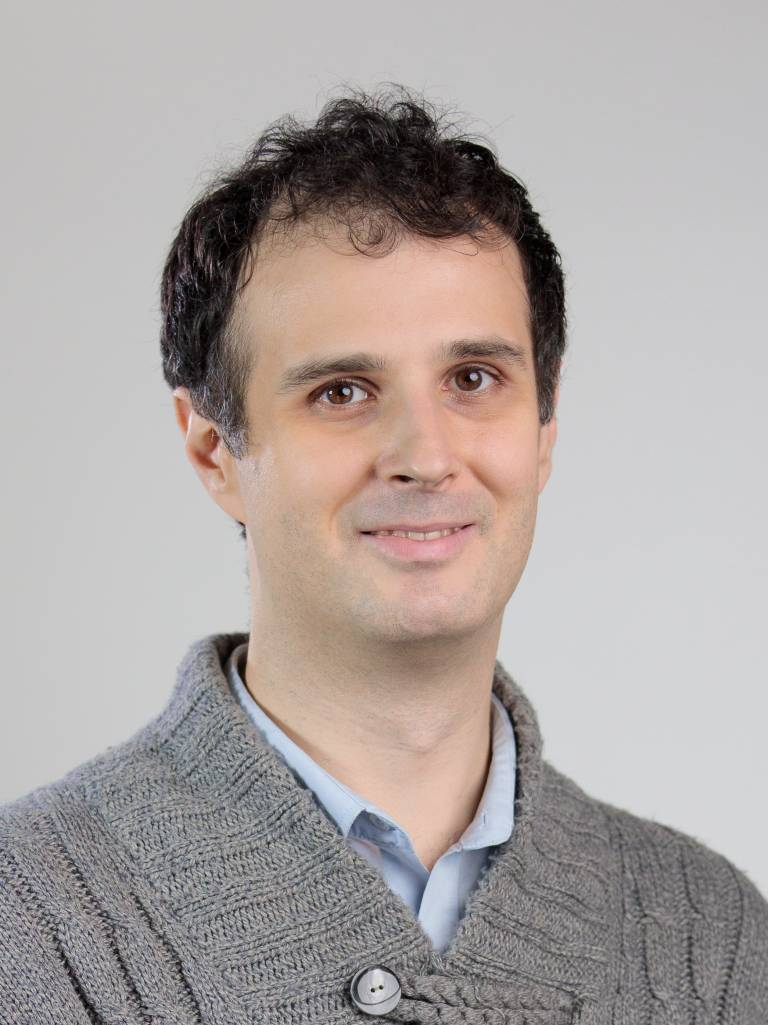Local Algorithms on Sparse Random Graphs Research Group

Head: Endre Csóka, Alfréd Rényi Institute of Mathematics

We examine the structures and phase transitions in large random graphs. For example, the structure of a largest independent set in a random $$d$$-regular graph on $$n → ∞$$ vertices is in a "solid state" for $$d ≥ 20$$ but probably in a "spin glass state" for $$d ≤ 19$$ . We are using the tools of graph limit theory, local algorithms (IID-factor processes), and probability theory, and we are trying to translate and extend some intuitive arguments in statistical physics into this mathematical language.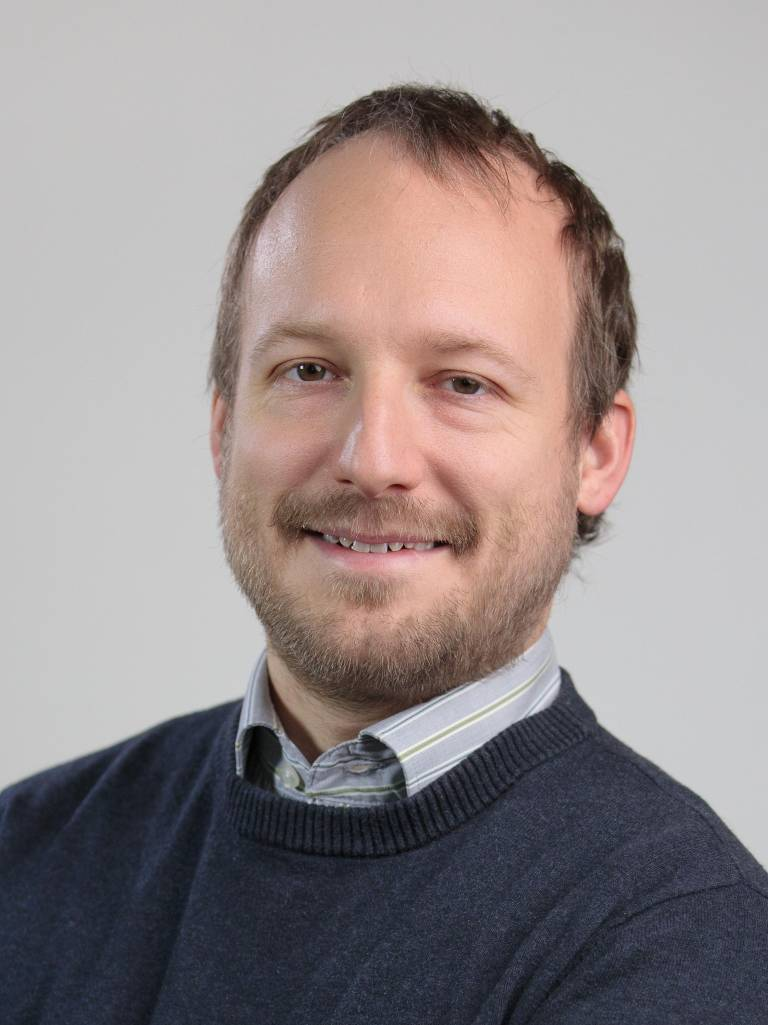Analysis and Applications of Markov Chains Research Group

Head: Balázs Gerencsér, Alfréd Rényi Institute of Mathematics

Our group is analyzing and exploring favorable perturbations of Markov chain transition probabilities towards nding the optimally mixing instance, also investigating minor changes in the transition topology (mostly discrete time chains). Similar research is carried out on a parallel track with an applied mind, in particular for consensus on networked systems, taking into account possibly asynchronous communication and similar challenges.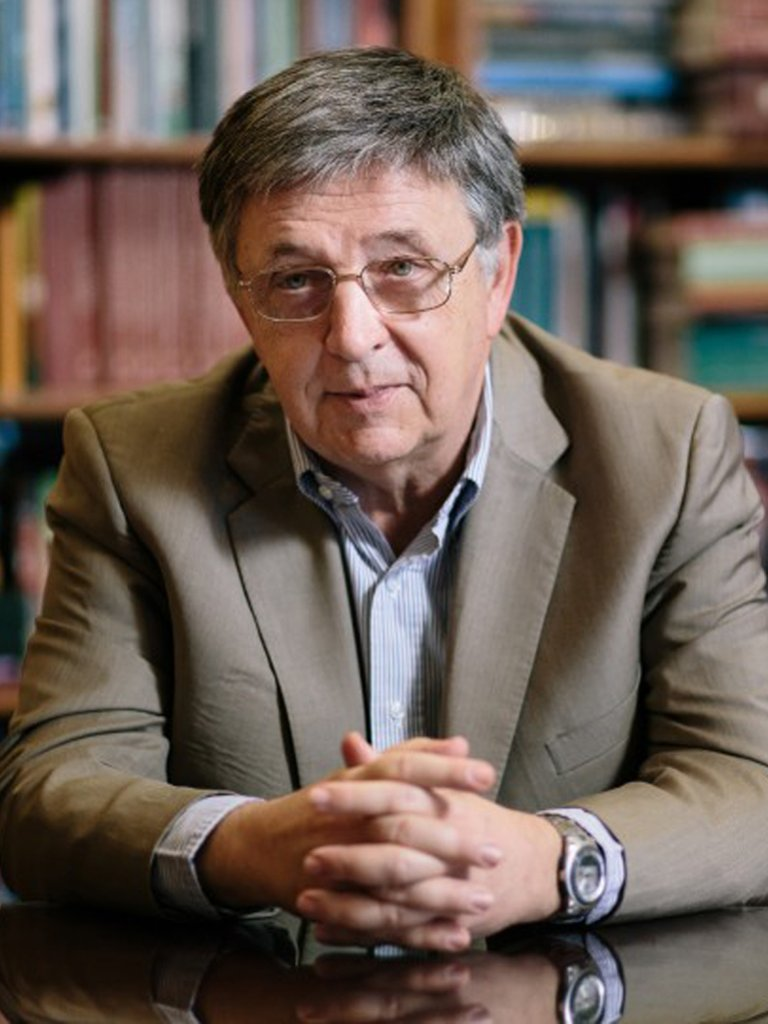Dynamics and Structure of Networks Research Group

Head: László Lovász, Alfréd Rényi Institute of Mathematics

A lot is known about the structure of large networks (graphs), but most real-life networks are interesting because they support interesting dynamics. Our research group is working on extending the structure theory of large networks to the case of graphs with medium density and to graphs supporting dynamics. Motivation comes from graph limit theory, epidemic propagation, and networks embedded in physical space.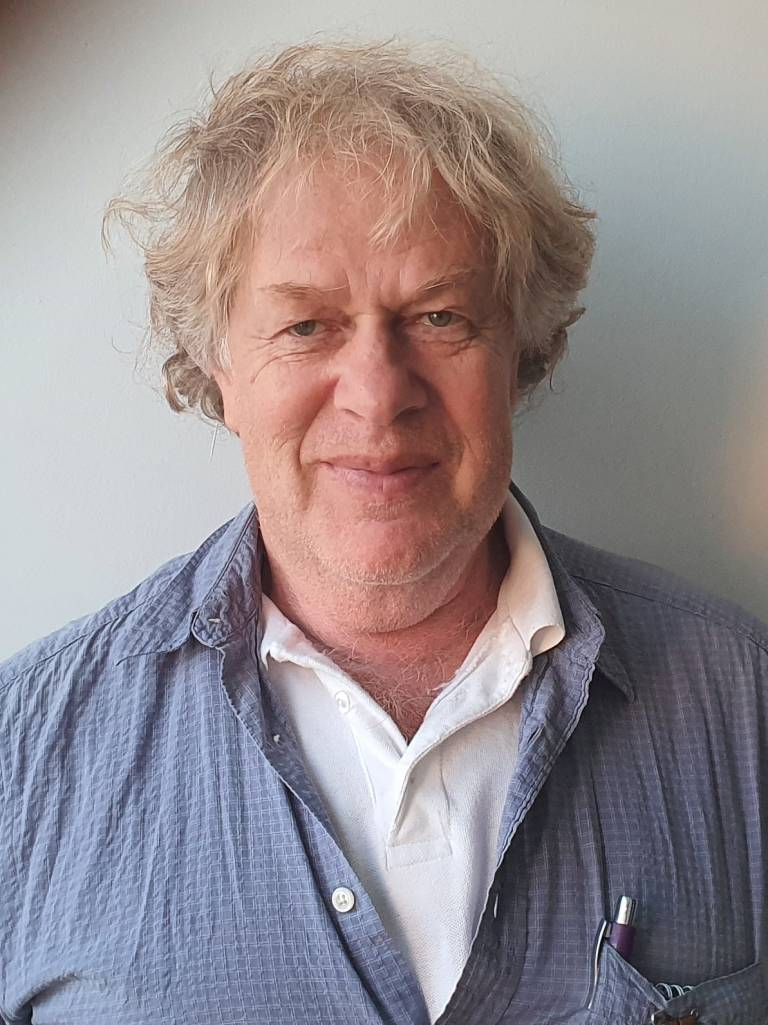GeoScape: Combinatorial Geometry Research Group

Head: János Pach, Alfréd Rényi Institute of Mathematics

A classic theme of combinatorics is to search for interesting patterns in various graphs and hypergraphs. Much of the research in Ramsey-theory, Turán-type extremal graph theory, Szemerédi regularity theory found important applications in combinatorial and computational geometry. However, if we blindly apply these theories in geometric scenarios, the obtained results are rarely optimal. We try to explain this curious phenomenon by proving stronger results on structures often arising in geometric applications: graphs and hypergraphs of bounded complexity, containing no "forbidden" subpattern of a fixed type, or embedded in a surface.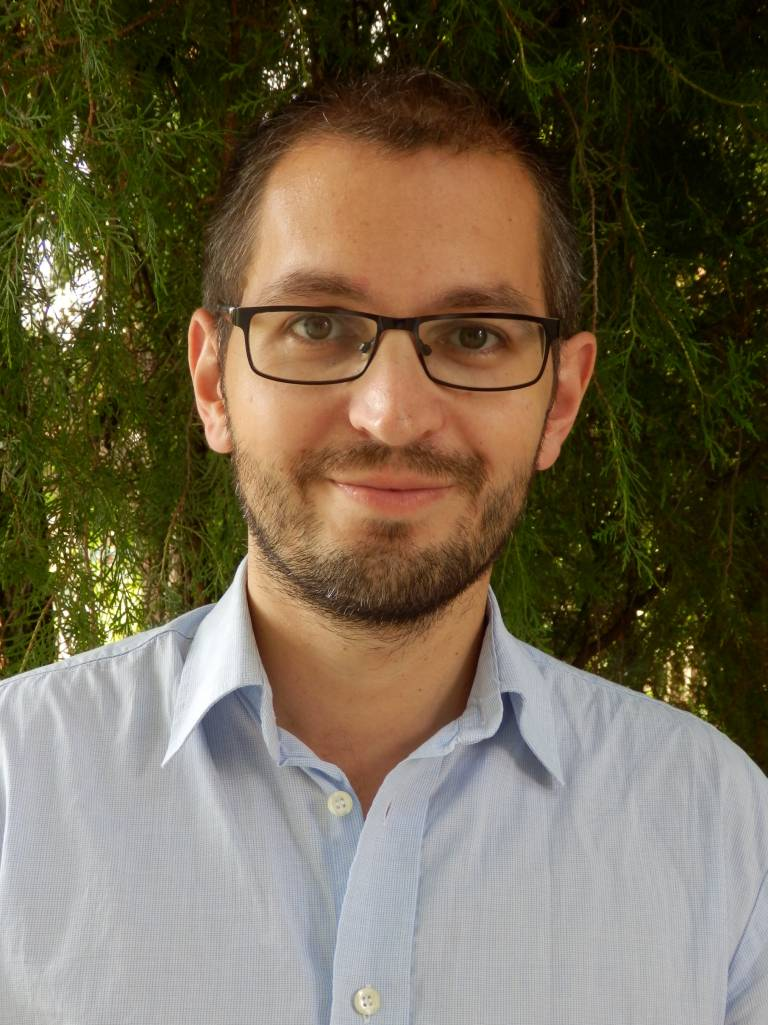MTA-BME Momentum Arithmetic Combinatorics Research Group

Head: Péter Pál Pach, Budapest University of Technology and Economics

Our aim is to introduce novel methods to solve problems of various types from Arithmetic Combinatorics. We particularly concentrate on developing techniques built on (linear) algebraic grounds like the slice rank method. A central question to be investigated is the following: What is the largest possible size of a k-AP-free subset of $$\mathbb{Z}_{p}^{n}$$ ?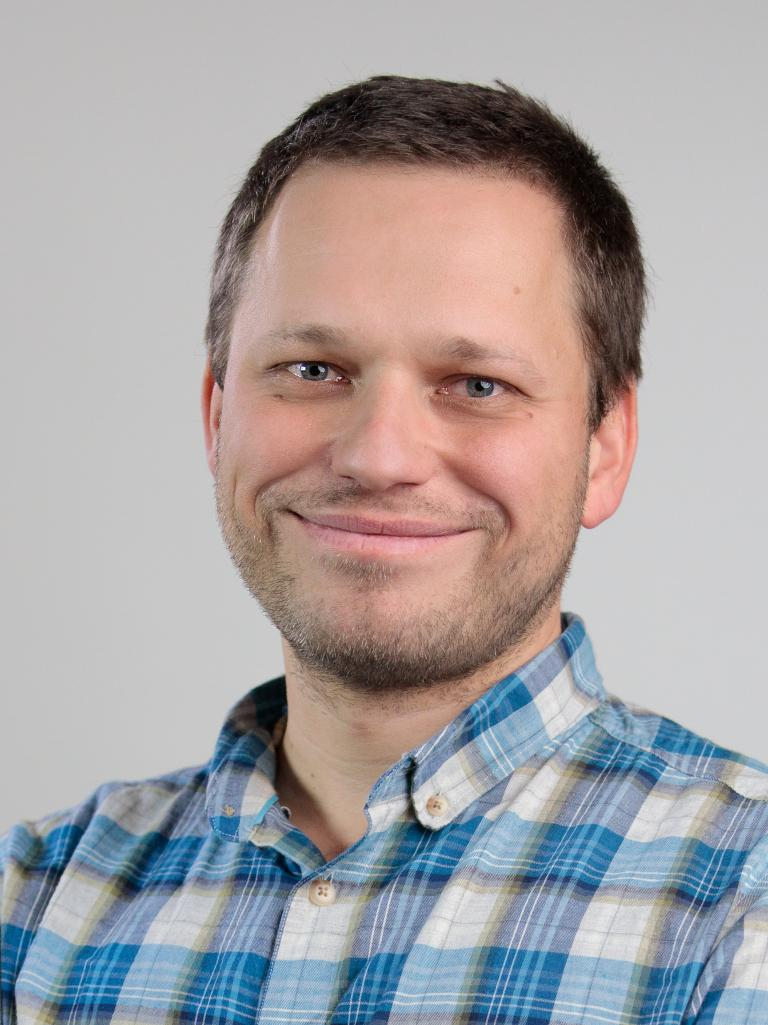Noise-Sensitivity Everywhere Research Group

Head: Gábor Pete, Alfréd Rényi Institute of Mathematics and Budapest University of Technology and Economics

Noise-sensitivity of a Boolean function with random input bits means that resampling a tiny proportion of the input makes the output decorrelate faster than the relaxation time of the entire system. For iid random input, discrete Fourier analysis yields a useful theory to understand what functions are noise-sensitive, with great applications, e.g., in percolation theory. What happens for non-iid input, such as the Ising model, Gaussian random fields, spanning forests, or factor of iid processes? A related goal is to study the (near-)critical behavior of statistical physics models on Cayley graphs of groups and on random graphs.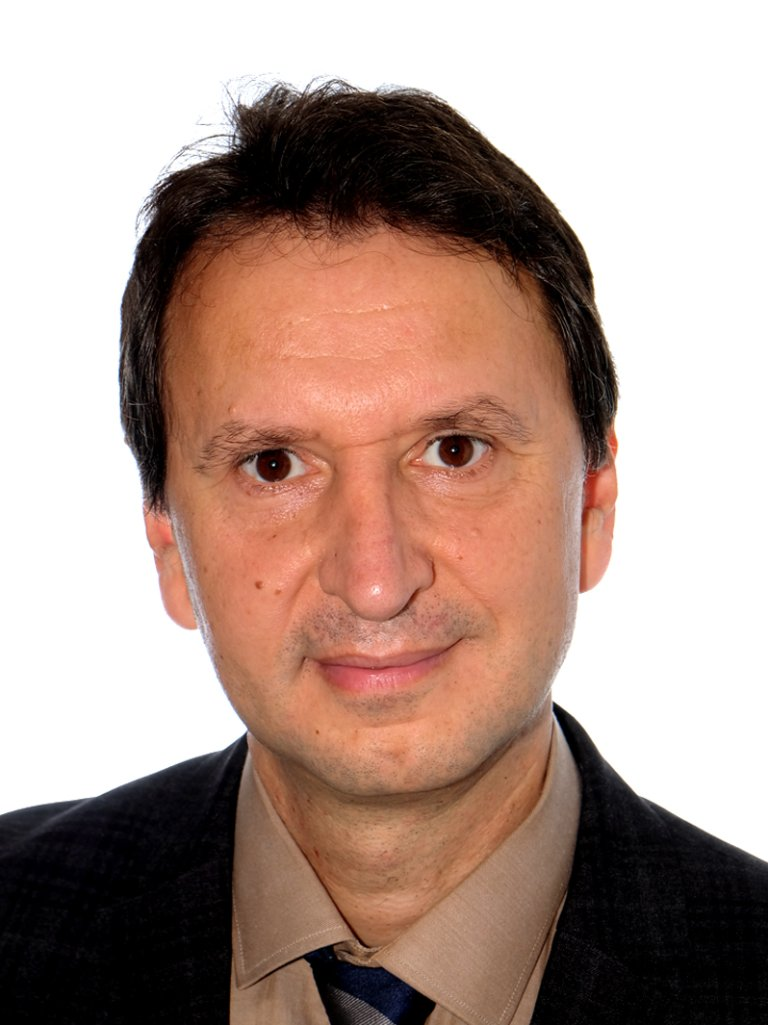Limits of Discrete Structures Research Group

Head: Balázs Szegedy, Alfréd Rényi Institute of Mathematics

We study large networks using methods from analysis, probability theory and algebra. More generally we develop limit concepts for various discrete structures.
Other closely related research areas include higher order Fourier analysis, dynamical systems and even machine learning.

Miklós Abért
Kristóf Bérczi
Péter Csikvári
Endre Csóka
Balázs Gerencsér
László Lovász
János Pach
Péter Pál Pach
Gábor Pete
Balázs Szegedy

### Participants

Gábor Damásdi
James Davies
John Fernley
Nóra Frankl
Partha Pratim Ghosh
Cedric Koh
Béla Rácz
Amedeo Sgueglia
Riley Thornton